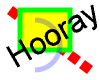## Python paint module

#### Examples

```import paint

image = paint.image(100, 80)
image.stroke(paint.line(10, 10, 90, 70).dash(0, (10, 5)), paint.rgb(0xff,0,0), 10)
rect = paint.rect(20, 10, 70, 50)
image.fill(rect, paint.rgb(0xff,0xff,0))
image.stroke(rect, paint.rgb(0,0xff,0), 5)
image.stroke(paint.arc(10, 20, 60, 70, 270, 180), paint.rgba(0,0,0xff,0x40), 5)
arial = paint.font('arial.ttf', 24, 30)
image.text(arial, 15, 70, paint.rgb(0,0,0), 'Hooray')
image.write_png('intro.png')
```
 Produces this imageClick for zoomed image showing libart / freetype anti-aliasing.

Step by step, the program does the following:

1. import paint
Loads the paint module so it can be used to produce images.
2. image = paint.image(100, 80)
Creates an image of width 100 pixels and height 80 pixels.
3. image.stroke(paint.line(10, 10, 90, 70).dash(0, (10, 5)), paint.rgb(0xff,0,0), 10)
Returns a vector path which describes a line from x=10,y=10 to x=90,y=70.
4. image.stroke(paint.line(10, 10, 90, 70).dash(0, (10, 5)), paint.rgb(0xff,0,0), 10)
Returns a dashed version of the previous line vector path which has 10 pixel dashes separated by 5 pixel gaps. The first dash starts at offset 0.
5. image.stroke(paint.line(10, 10, 90, 70).dash(0, (10, 5)), paint.rgb(0xff,0,0), 10)
Returns an RGBA color (red = 0xff, green = 0, blue = 0, alpha = 0xff) which is used to draw the dashed line.
6. image.stroke(paint.line(10, 10, 90, 70).dash(0, (10, 5)), paint.rgb(0xff,0,0), 10)
Draws a 10 pixel wide dashed line vector path in the specified color.
7. rect = paint.rect(20, 10, 70, 50)
Returns a vector path which describes a rectangle with the top-left corner at x=20,y=10 and bottom right corner at x=80,y=60.
8. image.fill(rect, paint.rgb(0xff,0xff,0))
Fills the rectangle vector path with yellow.
9. image.stroke(rect, paint.rgb(0,0xff,0), 5)
Draws a 5 pixel wide border around the rectangle vector path in green.
10. image.stroke(paint.arc(10, 20, 60, 70, 270, 180), paint.rgba(0,0,0xff,0x40), 5)
Returns a vector path which describes an arc starting at 270° and sweeping anti-clockwise through 180° from the ellipse bounded by the rectangle from with the top-left corner at x=10,y=20 and bottom right corner at x=60,y=70.
11. image.stroke(paint.arc(10, 20, 60, 70, 270, 180), paint.rgba(0,0,0xff,0x40), 5)
Returns an RGBA color (red = 0, green = 0, blue = 0xff, alpha = 0x40) which is used to draw the arc.
12. arial = paint.font('arial.ttf', 24, 30)
Loads the Arial TrueType font, scaled to 24 pixels and rotated 30°.
13. image.text(arial, 15, 70, paint.rgb(0,0,0), 'Hooray')
Draws the text 'Hooray' at x=15 y=70 in black.
14. image.write_png('intro.png')
Saves the image as a PNG file named intro.png